# R S Aggarwal Solutions for Class 11 Maths Chapter 21 Circles Exercise 21A

R S Aggarwal Solutions Class 11 Maths are given here for Chapter 21 – Circles exercise 21A. These exercise problems are based on the equation of a circle in standard form. The R S Aggarwal study material for Class 11 can help students discover new ways to solve difficult problems. Students can download R S Aggarwal chapter 21 solutions and practice more problems on Circle.

## Download PDF of R S Aggarwal Solutions for Class 11 Maths Chapter 21 Circles Exercise 21A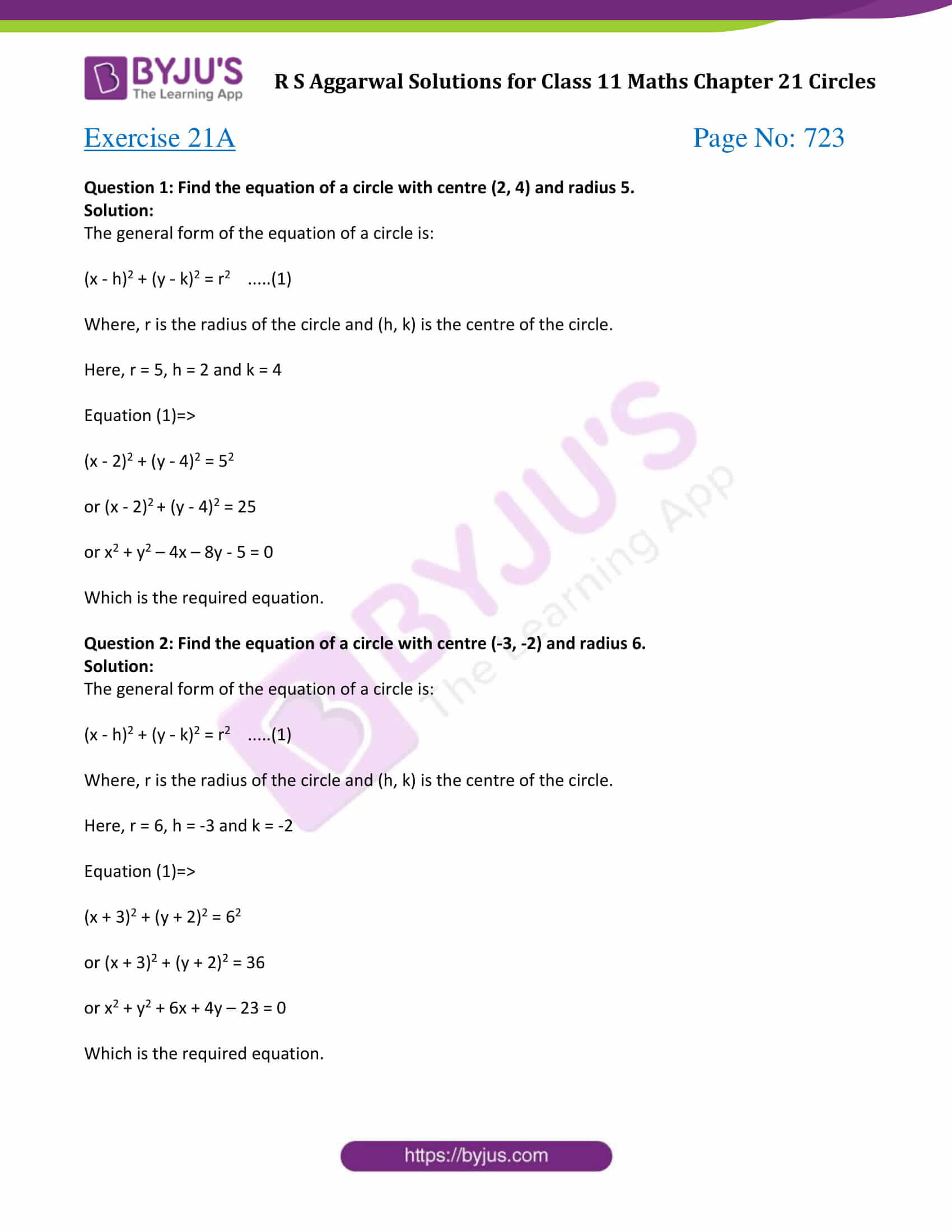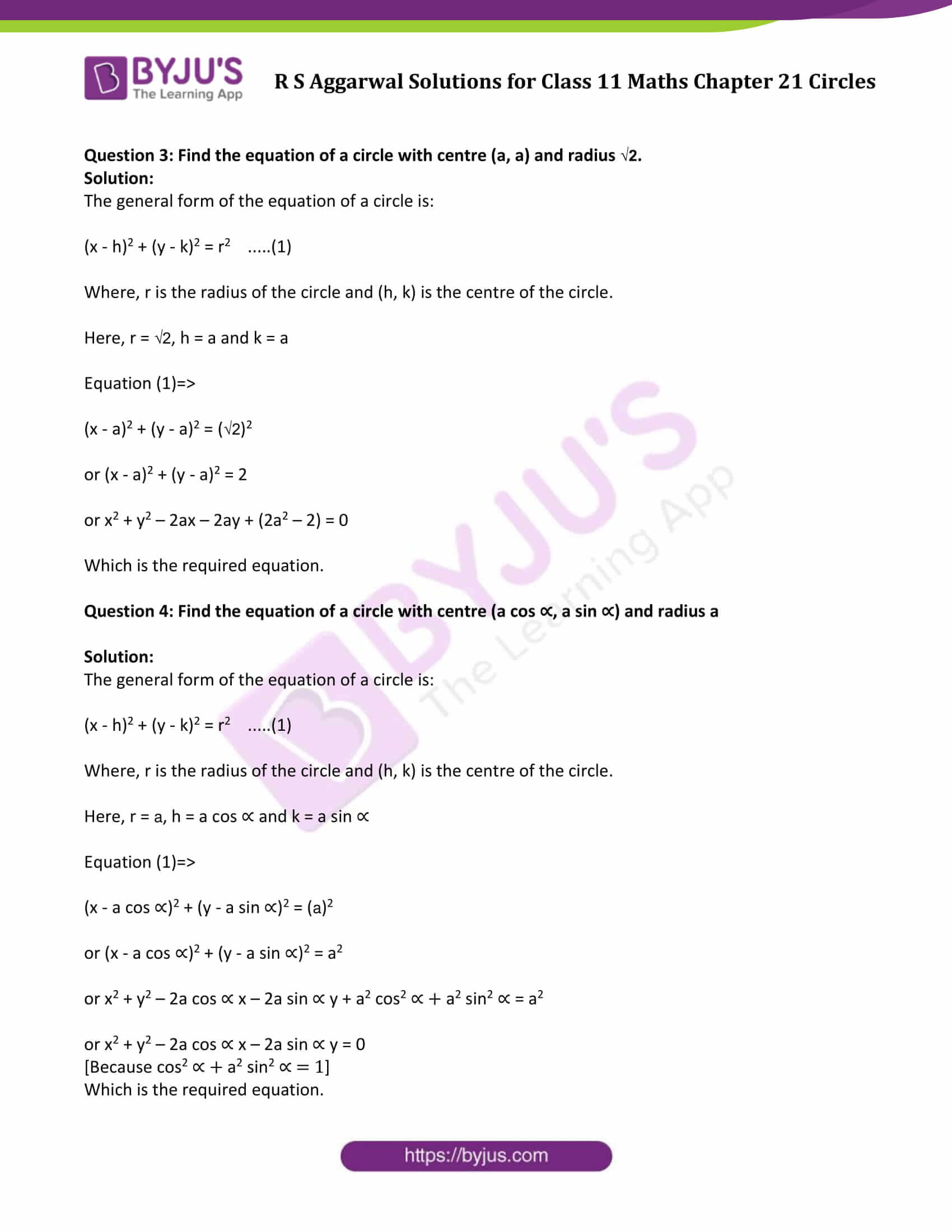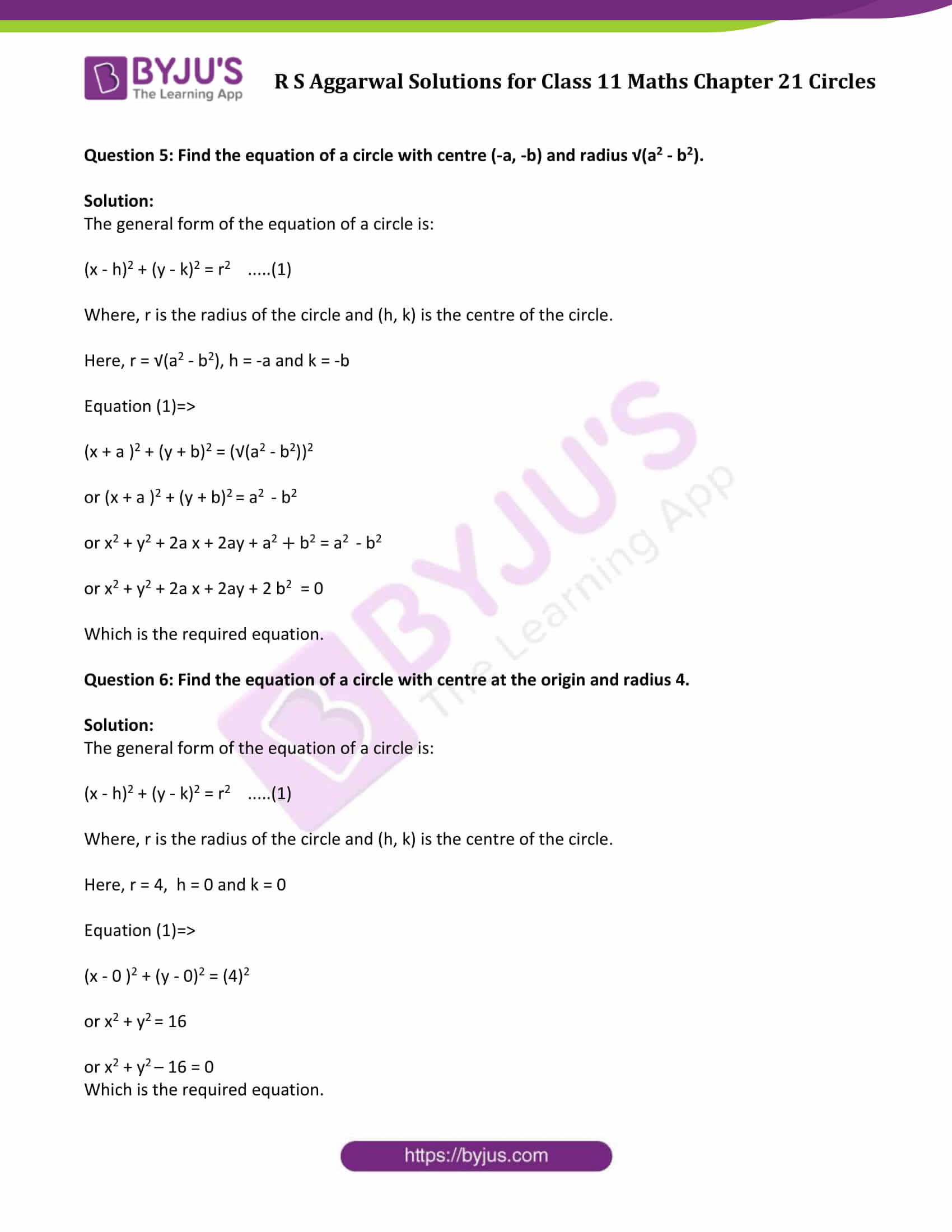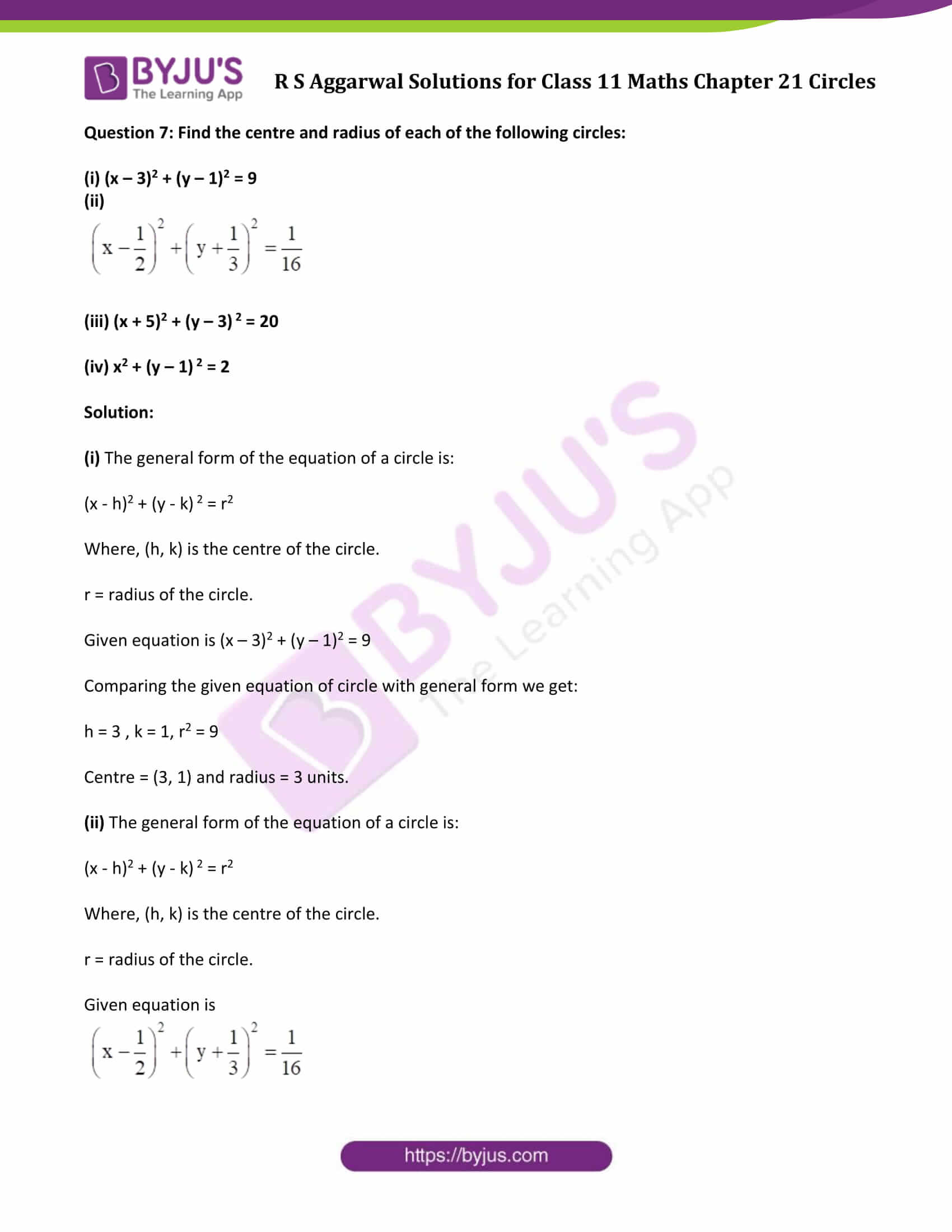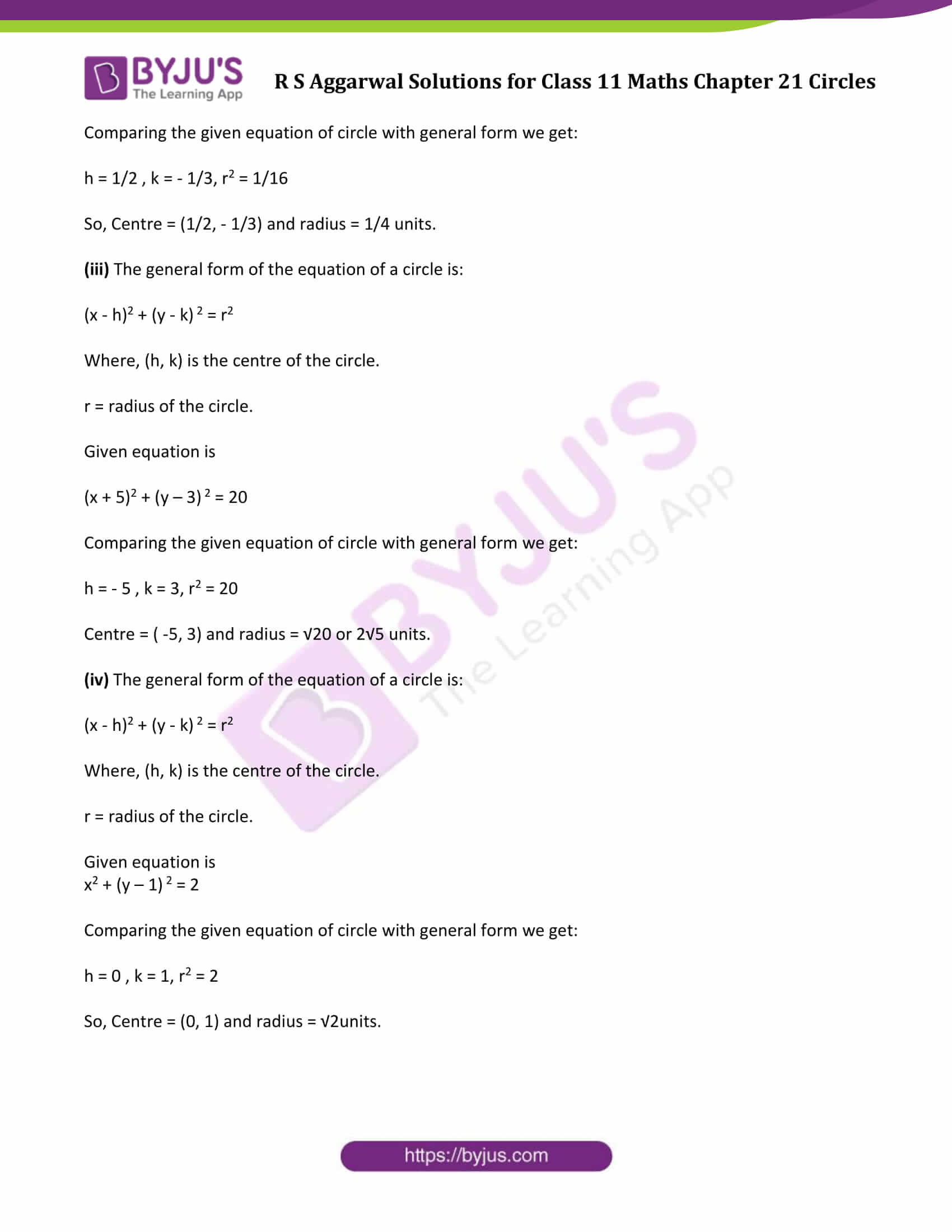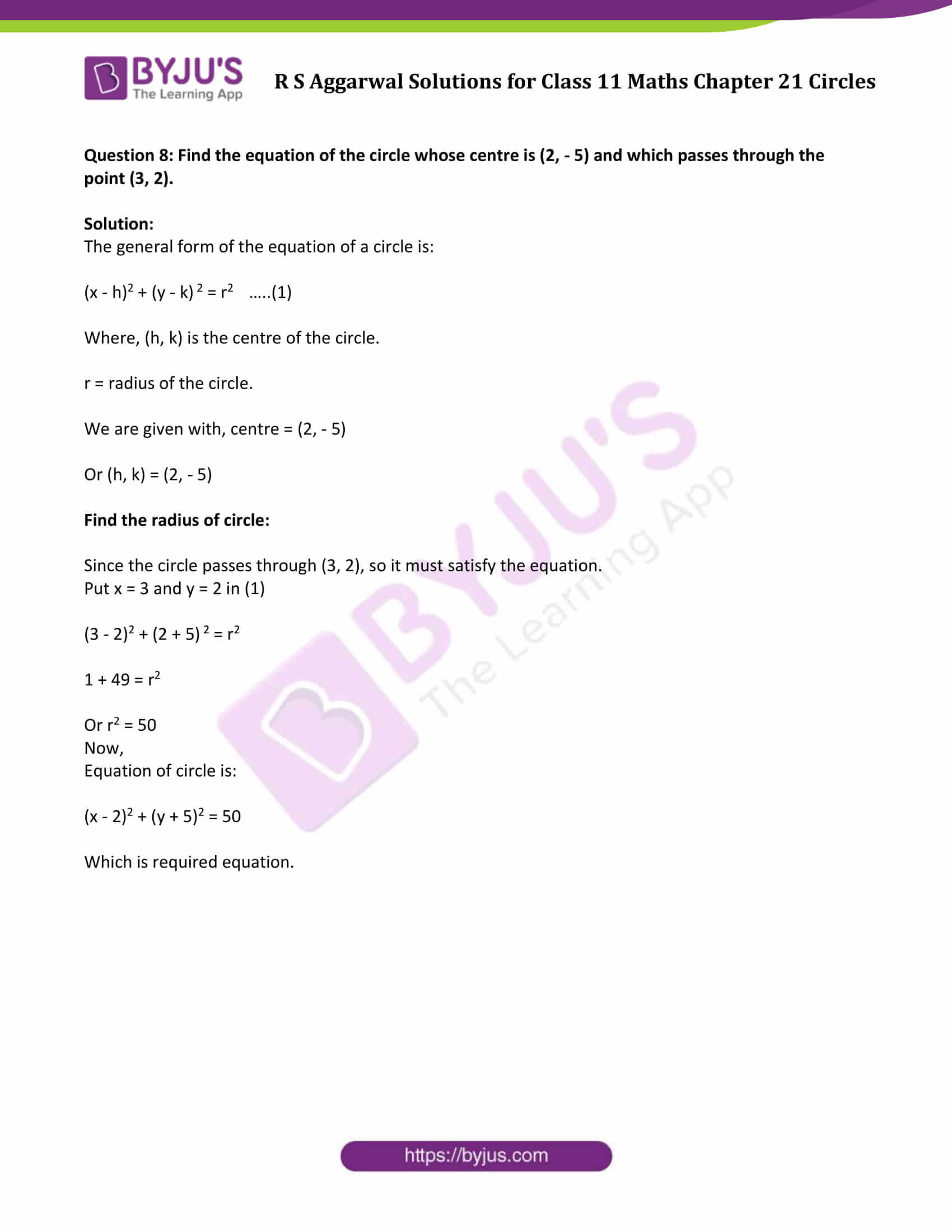### Access Answers of R S Aggarwal Solutions for Class 11 Maths Chapter 21 Circles Exercise 21A Page number 723

Exercise 21A Page No: 723

Question 1: Find the equation of a circle with centre (2, 4) and radius 5.

Solution:

The general form of the equation of a circle is:

(x – h)2 + (y – k)2 = r2 …..(1)

Where, r is the radius of the circle and (h, k) is the centre of the circle.

Here, r = 5, h = 2 and k = 4

Equation (1)⇒

(x – 2)2 + (y – 4)2 = 52

or (x – 2)2 + (y – 4)2 = 25

or x2 + y2 – 4x – 8y – 5 = 0

Which is the required equation.

Question 2: Find the equation of a circle with centre (-3, -2) and radius 6.

Solution:

The general form of the equation of a circle is:

(x – h)2 + (y – k)2 = r2 …..(1)

Where, r is the radius of the circle and (h, k) is the centre of the circle.

Here, r = 6, h = -3 and k = -2

Equation (1)⇒

(x + 3)2 + (y + 2)2 = 62

or (x + 3)2 + (y + 2)2 = 36

or x2 + y2 + 6x + 4y – 23 = 0

Which is the required equation.

Question 3: Find the equation of a circle with centre (a, a) and radius √2.

Solution:

The general form of the equation of a circle is:

(x – h)2 + (y – k)2 = r2 …..(1)

Where, r is the radius of the circle and (h, k) is the centre of the circle.

Here, r = √2, h = a and k = a

Equation (1)⇒

(x – a)2 + (y – a)2 = (√2)2

or (x – a)2 + (y – a)2 = 2

or x2 + y2 – 2ax – 2ay + (2a2 – 2) = 0

Which is the required equation.

Question 4: Find the equation of a circle with centre (a cos ∝, a sin ∝) and radius a

Solution:

The general form of the equation of a circle is:

(x – h)2 + (y – k)2 = r2 …..(1)

Where, r is the radius of the circle and (h, k) is the centre of the circle.

Here, r = a, h = a cos ∝ and k = a sin ∝

Equation (1)⇒

(x – a cos ∝)2 + (y – a sin ∝)2 = (a)2

or (x – a cos ∝)2 + (y – a sin ∝)2 = a2

or x2 + y2 – 2a cos ∝ x – 2a sin ∝ y + a2 cos2 ∝ + a2 sin2 ∝ = a2

or x2 + y2 – 2a cos ∝ x – 2a sin ∝ y = 0

[Because cos2 ∝ + a2 sin2 ∝ = 1]

Which is the required equation.

Question 5: Find the equation of a circle with centre (-a, -b) and radius √(a2 – b2).

Solution:

The general form of the equation of a circle is:

(x – h)2 + (y – k)2 = r2 …..(1)

Where, r is the radius of the circle and (h, k) is the centre of the circle.

Here, r = √(a2 – b2), h = -a and k = -b

Equation (1)⇒

(x + a )2 + (y + b)2 = (√(a2 – b2))2

or (x + a )2 + (y + b)2 = a2 – b2

or x2 + y2 + 2a x + 2ay + a2 + b2 = a2 – b2

or x2 + y2 + 2a x + 2ay + 2 b2 = 0

Which is the required equation.

Question 6: Find the equation of a circle with centre at the origin and radius 4.

Solution:

The general form of the equation of a circle is:

(x – h)2 + (y – k)2 = r2 …..(1)

Where, r is the radius of the circle and (h, k) is the centre of the circle.

Here, r = 4, h = 0 and k = 0

Equation (1)⇒

(x – 0 )2 + (y – 0)2 = (4)2

or x2 + y2 = 16

or x2 + y2 – 16 = 0

Which is the required equation.

Question 7: Find the centre and radius of each of the following circles:

(i) (x – 3)2 + (y – 1)2 = 9

(ii)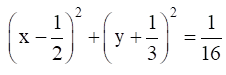(iii) (x + 5)2 + (y – 3) 2 = 20

(iv) x2 + (y – 1) 2 = 2

Solution:

(i) The general form of the equation of a circle is:

(x – h)2 + (y – k) 2 = r2

Where, (h, k) is the centre of the circle.

r = radius of the circle.

Given equation is (x – 3)2 + (y – 1)2 = 9

Comparing the given equation of circle with general form we get:

h = 3 , k = 1, r2 = 9

Centre = (3, 1) and radius = 3 units.

(ii) The general form of the equation of a circle is:

(x – h)2 + (y – k) 2 = r2

Where, (h, k) is the centre of the circle.

r = radius of the circle.

Given equation isComparing the given equation of circle with general form we get:

h = 1/2 , k = – 1/3, r2 = 1/16

So, Centre = (1/2, – 1/3) and radius = 1/4 units.

(iii) The general form of the equation of a circle is:

(x – h)2 + (y – k) 2 = r2

Where, (h, k) is the centre of the circle.

r = radius of the circle.

Given equation is

(x + 5)2 + (y – 3) 2 = 20

Comparing the given equation of circle with general form we get:

h = – 5 , k = 3, r2 = 20

Centre = ( -5, 3) and radius = √20 or 2√5 units.

(iv) The general form of the equation of a circle is:

(x – h)2 + (y – k) 2 = r2

Where, (h, k) is the centre of the circle.

r = radius of the circle.

Given equation is

x2 + (y – 1) 2 = 2

Comparing the given equation of circle with general form we get:

h = 0 , k = 1, r2 = 2

So, Centre = (0, 1) and radius = √2units.

Question 8: Find the equation of the circle whose centre is (2, – 5) and which passes through the point (3, 2).

Solution:

The general form of the equation of a circle is:

(x – h)2 + (y – k) 2 = r2 …..(1)

Where, (h, k) is the centre of the circle.

r = radius of the circle.

We are given with, centre = (2, – 5)

Or (h, k) = (2, – 5)

Since the circle passes through (3, 2), so it must satisfy the equation.

Put x = 3 and y = 2 in (1)

(3 – 2)2 + (2 + 5) 2 = r2

1 + 49 = r2

Or r2 = 50

Now,

Equation of circle is:

(x – 2)2 + (y + 5)2 = 50

Which is required equation.

## Access other exercise solutions of Class 11 Maths Chapter 21 Circles

Exercise 21B Solutions

## R S Aggarwal Solutions for Class 11 Maths Chapter 21 Exercise 21A

Class 11 Maths Chapter 21 Circles Exercise 21A is based on the following topics :

• Equation of a circle in standard form
• Equation of a circle, the end points of whose diameter are given